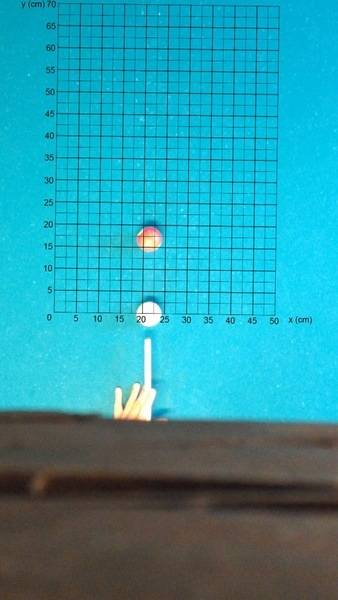# Velocity vector of cue ball

## Homework StatementI need to find the velocity vector of the cue ball. I know the start-coordinates, end-coordinates(when it hits the red ball), and the time it takes for the travel, but i have no clue on how to make the vector.
start(21, 0)
end(21, 12)
time = 0.1seconds
not sure if nessecary, but both of the balls diameters are 5.715cm

I need the velocity vector to calculate the end-coordinates of the red ball.

help is much appreciated :)